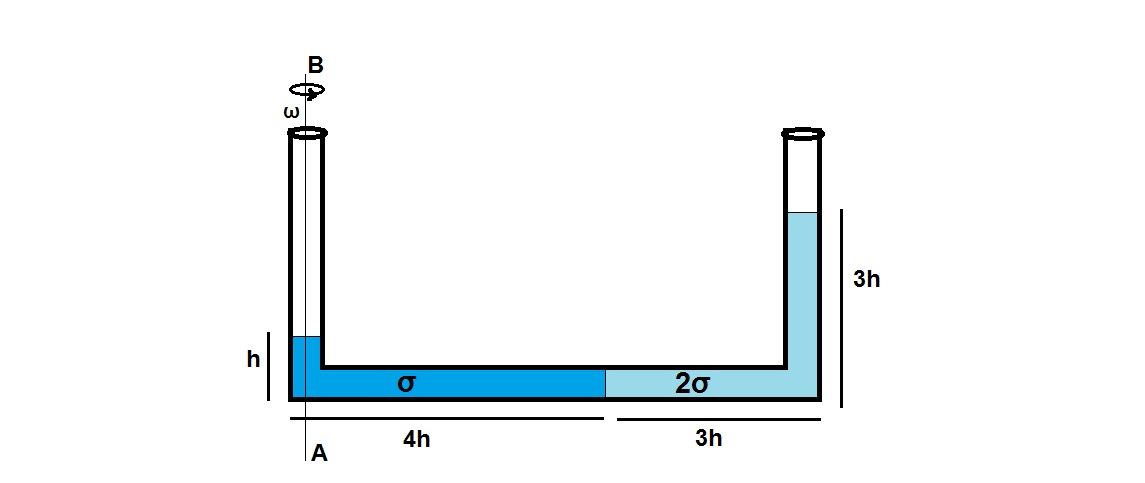# Rotating Vessel- Part IIThis Problem is a little harder than the previous version, "Rotating vessel". A U-Shaped vessel containing 2 liquids of varying densities σ and 2σ is rotated about the axis AB so that the arrangement in the picture takes place. The height of liquid of density σ is h and that of density 2σ is 3h. The whole horizontal length of the tube is 7h out of which 4h is occupied by liquid of density σ. Find the angular velocity ω with which the tube is rotated.

Note:
$g = 10 m/s^{2}$
$h = \frac{2}{41} m$

×